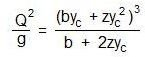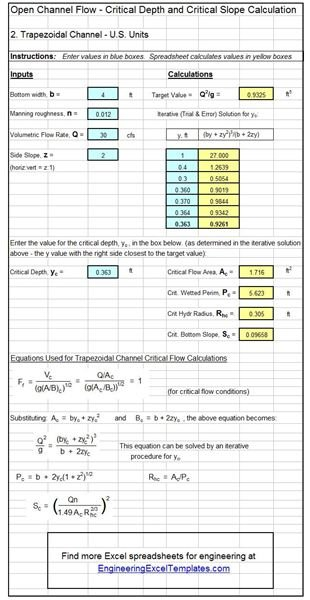# Froude Number and Manning Equation in Calculation of Critical Depth for Open Channel Flow

Page content

## Introduction to Critical, Subcritical and Supercritcal Flow

Any case of open channel flow must fit into one of the three categories: i) subcritical flow, ii) supercritical flow, or iii) critical flow. General characteristics of supercritical flow are high velocity and shallow flow, while subcritical flow is, in general, a deep, low velocity flow. Critical flow conditions divide supercritical and subcritical flow. The article, “Open Channel Flow Basics 2 - Supercritical Flow” has information on the theory behind critical, subcritical and supercritical flow and the relationship of the specific energy of a given flow to these three flow regimes. The hydraulic jump is an open channel flow phenomenon, in which knowledge about these three regimes of flow is important. The flow upstream of a hydraulic jump is always supercritical and the flow downstream of the jump is always subcritical. The second and third articles in this series (listed at the end of the article) give more details about hydraulic jumps and hydraulic jump calculations.

## Critical Flow Terminology

Knowledge of the following terminology is useful in discussing subcritical, supercritical, and critical flow calculations:

• The critical depth (yc**)** is the normal depth at critical flow conditions for a given flow rate in a given channel (i.e. given channel bottom slope, Manning roughness, and shape & size).
• The critical slope (Sc**)** is the channel bottom slope that will result in critical flow conditions for a given flow rate in a channel of given Manning roughness, size, and shape.
• The critical velocity (Vc**)** is the liquid velocity for critical flow conditions in a particular channel with specified flow rate.
• A channel carrying a specified flow rate has a steep slope if its slope is greater than the critical slope for that flow rate in that channel.
• A channel carrying a specified flow rate has a mild slope if its slope is less than the critical slope for that flow rate in that channel.

These parameters are shown in the diagram above right, which illustrates subcritical flow, critical flow, and supercritical flow.

## The Froude Number and Critical Open Channel Flow

The Froude number is of interest here because its value for any particular open channel flow provides information on whether that flow is subcritical, critical or supercritical flow. The Froude number is defined as follows: Fr = V/(gL)1/2, where

• Fr is the Froude Number
• V is the average velocity of the liquid in the channel (ft/sec for U.S. units or m/s for S.I.).
• g is the acceleration due to gravity (32.17 ft/sec2 for U.S. units or 9.81 m/s2 for S.I.).
• L is a characteristic length for the particular type of open channel (ft for U.S. units or m for S.I.)

For example, with flow in a rectangular channel, the characteristic length, L, is the depth of flow, y, making Fr = V/(gy)1/2 . For flow in a non-rectangular chanel, Fr = V/[g(A/B)]1/2, because the characteristic length, L, is A/B, where A is the cross-sectional area of liquid flow, and B is the surface width. For details on calculating cross-sectional area, surface width and hydraulic radius for several channel shapes, see the article, “Calculation of Hydraulic Radius for Open Channel flow.” As mentioned above, the value of the Froude number gives information about the type of flow. Details are summarized below:

• If Fr > 1, then it is supercritical flow
• If Fr < 1, then it is subcritical flow
• If Fr = 1, then it is critical flow

## Critical Depth and Critical Slope for a Rectangular Channel

The critical depth for a rectangular channel can be calculated from the fact that the Froude number is equal to one at critical flow conditions. For a rectangular channel , this fact leads to the equation: Vc/(gyc)1/2 = 1, where the subscript c is used to indicate critical flow conditions for the velocity and depth. Using the relationship that V = Q/A and that A = yb for a rectangular channel ( b = the channel width ), the equation above becomes: (Q/ycb)/(gyc)1/2 = 1. Solving for yc and substituting q = Q/b, leads to the following equation for the critical depth in a rectangular channel: yc = (q2**/g)**1/3. Thus, the only parameters needed to calculate the critical depth are the flow rate and the rectangular channel width.

After the critical depth is known, the critical slope can be calculated from the Manning equation. Solving for slope in the Manning equation written for critical flow conditions leads to the following equation for the critical slope: Sc = [Qn/(1.49AcRhc2/3)]2. Note that the critical hydraulic radius, (Rhc) is the critical area (Ac) divided by the critical wetted perimeter (Pc), where Ac = byc and Pc = b + 2yc. The Manning equation is a dimensional equation, requiring the following units for the variables: Q in cfs, Ac in ft2 , and Rhc in ft. Sc and n are both dimensionless.

## Calculations for a Trapezoidal ChannelFor a channel with a trapezoidal cross section, the critical flow condition is given by Fr = Vc/[g(A/B)c]1/2 = 1, where Ac = yc(b + zyc) and Bc = b + zyc2 , where z is the trapezoidal channel side slope (H:V = z:1). Substituting values into the Fr =1 equation leads to the equation at the right, which has yc as the only unknown. This equation requires an iterative solution, because it can’t be solved explicitly for yc.

Determination of critical slope for a trapezoidal channel uses the same equation given above for a rectangular channel, with the expression above for Ac , Pc = 2yc(1 + z2)1/2 , and Rhc = Ac/Pc.

## Use of S.I. Units

All of the equations except the Manning equation remain the same for S.I. units. In the Manning equation, the 1.49 constant becomes 1.00, giving the following equation for critical slope: Sc = [Qn/(1.00AcRhc2/3)]2. In this equation, Q must be in m3/s, Ac in m2 , and Rhc in m.

## Example CalculationConsider a trapezoidal channel with bottom width (b) = 4 ft, Manning roughness coefficient (n) = 0.012, side slope (z) = 2, carrying 30 cfs. What would be the critical depth and the critical slope for this flow rate in this channel?

Solution: The diagram at the right shows a spreadsheet solution of this calculation. An iterative solution of the equation, Q2/g = (byc + zyc2)3/(b + 2zyc) give: yc = 0.363 ft.

Calculation of the critical slope using the equation, Sc = [Qn/(1.49AcRhc2/3)]2, shows: Sc = 0.09658.

1. Munson, B. R., Young, D. F., & Okiishi, T. H., Fundamentals of Fluid Mechanics, 4th Ed., New York: John Wiley and Sons, Inc, 2002.

2. Chow, V. T., Open Channel Hydraulics, New York: McGraw-Hill, 1959.

3. Bengtson, Harlan H., Open Channel Flow Spreadsheets - Critical Depth and Critical Slope.

4. Bengtson, Harlan H., Open Channel Flow II - Hydraulic Jumps and Supercritical and Nonuniform Flow - An online, continuing education course for PDH credit.

## This post is part of the series: Open Channel Flow Basics

Open channel flow takes place with a free surface open to the atmosphere, so flow is due to gravity, rather than due to pressure as in pipe flow. The Manning equation applies for uniform flow. A hydraulic jump is nonuniform flow. It occurs for supercritical flow on a subcritical channel slope.